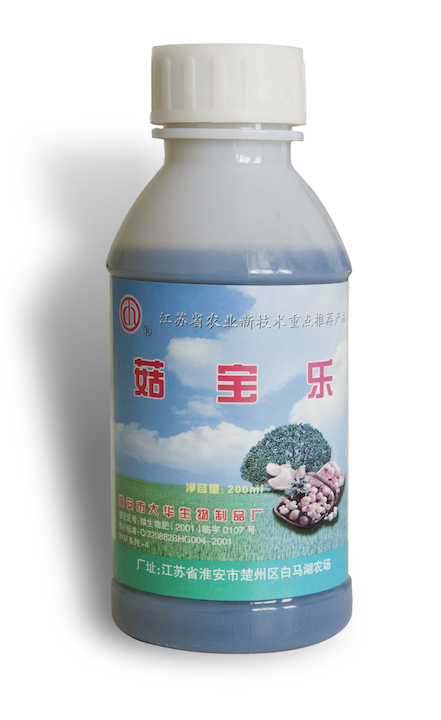• 水产专用
• 大量元素水溶肥料
• 含腐殖酸水溶肥料
• 含氨基酸水溶肥料
• 发酵菌剂
• 沼气发酵菌剂
• 肥水专用
• 净水剂
• 发酵增产剂
• 生物反应堆菌剂
• 秸秆饲料发酵剂
• 酵素菌速腐剂
• 废菌料发酵剂
• 育苗基质
• 水稻育苗基质
• 蓝莓栽培基质
• 蔬菜育苗基质
• 生物有机肥
• 有机肥料
• 生物有机肥
• 食用菌专用
• 发酵增产剂
• 菇宝乐
• 联系我们
• 产品分类
• 发酵菌剂
• 生物反应堆菌剂
• 沼气发酵菌剂
• 肥水专用
• 发酵增产剂
• 秸秆饲料发酵剂
• 废菌料发酵剂
• 酵素菌速腐剂
• 净水剂
• 水溶肥料
• 大量元素水溶肥料
• 含腐殖酸水溶肥料
• 含氨基酸水溶肥料
• 育苗基质
• 蓝莓栽培基质
• 蔬菜育苗基质
• 水稻育苗基质
• 生物有机肥
• 有机肥料
• 生物有机肥
• 水产制剂
• 水产专用
• 食用菌专用
• 菇宝乐
• 发酵增产剂
• 园艺资材
• 有机肥料
• 水稻育苗基质
• 花盆
• 含氨基酸水溶肥料
• 菇宝乐
• 发酵增产剂
• 土壤修复剂
• 土壤调理剂
• 产品分类
• 发酵菌剂
• 水溶肥料
• 育苗基质
• 生物有机肥
• 食用菌专用
• 园艺资材
• 土壤调理剂
• 土壤修复剂
• 水产制剂
• 产品分类
• 土壤调理剂
• 土壤修复剂
• 水产制剂
• 水产专用
• 育苗基质
• 蓝莓栽培基质
• 蔬菜育苗基质
• 水稻育苗基质
• 发酵菌剂
• 发酵增产剂
• 肥水专用
• 沼气发酵菌剂
• 净水剂
• 生物反应堆菌剂
• 秸秆饲料发酵剂
• 废菌料发酵剂
• 酵素菌速腐剂
• 水溶肥料
• 大量元素水溶肥料
• 含氨基酸水溶肥料
• 含腐殖酸水溶肥料
• 生物有机肥
• 有机肥料
• 生物有机肥
• 食用菌专用
• 菇宝乐
• 发酵增产剂
• 园艺资材
• 菇宝乐
• 发酵增产剂
• 花盆
• 水稻育苗基质
• 含氨基酸水溶肥料
• 有机肥料
• 产品分类
• 发酵菌剂
• 发酵增产剂
• 沼气发酵菌剂
• 净水剂
• 生物反应堆菌剂
• 秸秆饲料发酵剂
• 废菌料发酵剂
• 酵素菌速腐剂
• 肥水专用
• 水溶肥料
• 大量元素水溶肥料
• 含腐殖酸水溶肥料
• 含氨基酸水溶肥料
• 育苗基质
• 蓝莓栽培基质
• 蔬菜育苗基质
• 水稻育苗基质
• 生物有机肥
• 有机肥料
• 生物有机肥
• 土壤调理剂
• 食用菌专用
• 菇宝乐
• 发酵增产剂
• 园艺资材
• 有机肥料
• 水稻育苗基质
• 花盆
• 含氨基酸水溶肥料
• 发酵增产剂
• 菇宝乐
• 土壤修复剂
• 水产制剂
• 水产专用
• 产品分类
• 土壤调理剂
• 土壤修复剂
• 水产制剂
• 水产专用
• 发酵菌剂
• 沼气发酵菌剂
• 肥水专用
• 净水剂
• 发酵增产剂
• 生物反应堆菌剂
• 秸秆饲料发酵剂
• 废菌料发酵剂
• 酵素菌速腐剂
• 水溶肥料
• 含氨基酸水溶肥料
• 含腐殖酸水溶肥料
• 大量元素水溶肥料
• 育苗基质
• 蓝莓栽培基质
• 蔬菜育苗基质
• 水稻育苗基质
• 生物有机肥
• 有机肥料
• 生物有机肥
• 食用菌专用
• 菇宝乐
• 发酵增产剂
• 园艺资材
• 含氨基酸水溶肥料
• 菇宝乐
• 发酵增产剂
• 水稻育苗基质
• 有机肥料
• 花盆
• 网站首页
• 关于我们
• 总经理致辞
• 企业文化
• 组织架构
• 公司概况
• 产品分类
• 土壤调理剂
• 水产制剂
• 水产专用
• 园艺资材
• 有机肥料
• 水稻育苗基质
• 花盆
• 含氨基酸水溶肥料
• 菇宝乐
• 发酵增产剂
• 育苗基质
• 蓝莓栽培基质
• 蔬菜育苗基质
• 水稻育苗基质
• 生物有机肥
• 有机肥料
• 生物有机肥
• 食用菌专用
• 发酵增产剂
• 菇宝乐
• 土壤修复剂
• 水溶肥料
• 大量元素水溶肥料
• 含腐殖酸水溶肥料
• 含氨基酸水溶肥料
• 发酵菌剂
• 废菌料发酵剂
• 酵素菌速腐剂
• 沼气发酵菌剂
• 肥水专用
• 净水剂
• 发酵增产剂
• 生物反应堆菌剂
• 秸秆饲料发酵剂
• 产品分类
• 发酵菌剂
• 沼气发酵菌剂
• 肥水专用
• 净水剂
• 发酵增产剂
• 生物反应堆菌剂
• 秸秆饲料发酵剂
• 废菌料发酵剂
• 酵素菌速腐剂
• 水溶肥料
• 大量元素水溶肥料
• 含腐殖酸水溶肥料
• 含氨基酸水溶肥料
• 育苗基质
• 蓝莓栽培基质
• 蔬菜育苗基质
• 水稻育苗基质
• 生物有机肥
• 有机肥料
• 生物有机肥
• 食用菌专用
• 菇宝乐
• 发酵增产剂
• 园艺资材
• 含氨基酸水溶肥料
• 有机肥料
• 花盆
• 菇宝乐
• 发酵增产剂
• 水稻育苗基质
• 土壤调理剂
• 土壤修复剂
• 水产制剂
• 水产专用
• 产品分类
• 园艺资材
• 有机肥料
• 水稻育苗基质
• 花盆
• 含氨基酸水溶肥料
• 菇宝乐
• 发酵增产剂
• 土壤调理剂
• 水产制剂
• 水产专用
• 土壤修复剂
• 发酵菌剂
• 生物反应堆菌剂
• 沼气发酵菌剂
• 肥水专用
• 发酵增产剂
• 秸秆饲料发酵剂
• 废菌料发酵剂
• 酵素菌速腐剂
• 净水剂
• 食用菌专用
• 菇宝乐
• 发酵增产剂
• 水溶肥料
• 大量元素水溶肥料
• 含腐殖酸水溶肥料
• 含氨基酸水溶肥料
• 育苗基质
• 蓝莓栽培基质
• 蔬菜育苗基质
• 水稻育苗基质
• 生物有机肥
• 生物有机肥
• 有机肥料
• 产品分类
• 食用菌专用
• 菇宝乐
• 发酵增产剂
• 水溶肥料
• 大量元素水溶肥料
• 含腐殖酸水溶肥料
• 含氨基酸水溶肥料
• 育苗基质
• 蓝莓栽培基质
• 蔬菜育苗基质
• 水稻育苗基质
• 生物有机肥
• 生物有机肥
• 有机肥料
• 园艺资材
• 有机肥料
• 水稻育苗基质
• 花盆
• 含氨基酸水溶肥料
• 菇宝乐
• 发酵增产剂
• 土壤调理剂
• 土壤修复剂
• 水产制剂
• 水产专用
• 发酵菌剂
• 废菌料发酵剂
• 酵素菌速腐剂
• 发酵增产剂
• 秸秆饲料发酵剂
• 生物反应堆菌剂
• 沼气发酵菌剂
• 肥水专用
• 净水剂
• 产品分类
• 发酵菌剂
• 沼气发酵菌剂
• 肥水专用
• 生物反应堆菌剂
• 净水剂
• 秸秆饲料发酵剂
• 废菌料发酵剂
• 酵素菌速腐剂
• 发酵增产剂
• 土壤修复剂
• 水溶肥料
• 大量元素水溶肥料
• 含腐殖酸水溶肥料
• 含氨基酸水溶肥料
• 育苗基质
• 蓝莓栽培基质
• 蔬菜育苗基质
• 水稻育苗基质
• 水产制剂
• 水产专用
• 食用菌专用
• 菇宝乐
• 发酵增产剂
• 园艺资材
• 有机肥料
• 水稻育苗基质
• 花盆
• 含氨基酸水溶肥料
• 菇宝乐
• 发酵增产剂
• 土壤调理剂
• 生物有机肥
• 生物有机肥
• 有机肥料
• 产品分类
• 育苗基质
• 蓝莓栽培基质
• 蔬菜育苗基质
• 水稻育苗基质
• 生物有机肥
• 有机肥料
• 生物有机肥
• 食用菌专用
• 菇宝乐
• 发酵增产剂
• 土壤调理剂
• 土壤修复剂
• 水产制剂
• 水产专用
• 园艺资材
• 水稻育苗基质
• 有机肥料
• 花盆
• 含氨基酸水溶肥料
• 菇宝乐
• 发酵增产剂
• 发酵菌剂
• 沼气发酵菌剂
• 肥水专用
• 净水剂
• 发酵增产剂
• 生物反应堆菌剂
• 秸秆饲料发酵剂
• 废菌料发酵剂
• 酵素菌速腐剂
• 水溶肥料
• 含腐殖酸水溶肥料
• 大量元素水溶肥料
• 含氨基酸水溶肥料
• 产品分类
• 土壤调理剂
• 土壤修复剂
• 水产制剂
• 水产专用
• 发酵菌剂
• 沼气发酵菌剂
• 肥水专用
• 净水剂
• 发酵增产剂
• 生物反应堆菌剂
• 秸秆饲料发酵剂
• 废菌料发酵剂
• 酵素菌速腐剂
• 水溶肥料
• 含氨基酸水溶肥料
• 含腐殖酸水溶肥料
• 大量元素水溶肥料
• 育苗基质
• 蓝莓栽培基质
• 蔬菜育苗基质
• 水稻育苗基质
• 生物有机肥
• 有机肥料
• 生物有机肥
• 食用菌专用
• 菇宝乐
• 发酵增产剂
• 园艺资材
• 花盆
• 菇宝乐
• 发酵增产剂
• 水稻育苗基质
• 含氨基酸水溶肥料
• 有机肥料
• ### 菇宝乐产品名称： 菇宝乐

发布日期： 2016-07-26

点击数： 1372

询价：

二维码：简述：

### 产品详情

菇宝乐是采用生物技术研制开发的菇类专用天然营养液。，内含天然活性葡萄糖、果糖、核糖、多种活性酶类、氨基酸以及菇耳类生长需要的多种维生素、微量元素，适用于各种食（药）用菌的拌料、喷施、补水、灌渗等，是生产绿色、有机食用菌产品的必备佳品。

产品功效
① 增加产量：促进食用菌菌丝及子实体对营养的吸收，以及菇盖的增大、增厚，产量可增加10%~30%
② 减轻病害：能有效抑制杂菌、预防疾病、降低污染率。
③ 改善品质 ：本产品所含的活性因子能促进子实体的形成及生长，改善菇体品质。
④ 提早出菇期：有缩短转潮期、活化板块的奇效功能，可提早出菇3~10天。
⑤ 消除药残：能消除因使用农药带来的药物残留，且无任何副作用。
使用方法

本产品可用于拌料、喷施、浸泡、注射、灌渗。①拌料：将本品稀释1000倍液用于生料栽培食用菌的原料拌料（熟料除外）;②喷施：用300~400倍液自幼菇期起每隔2~3天喷一次，连喷3次，直接喷施菇体;③浸泡：主要用于香菇代料栽培的后期浸水，在每潮菇采收后或菌袋放入容器内，用1000倍液浸泡；④注射：用500倍液注入菌棒内，补充养分不足；⑤灌渗：适用于床栽或畦载，用1000倍液从培养基上部均匀灌入。

版权所有：淮安亚博体育 登录生物科技有限公司
技术支持：仕德伟科技 苏ICP备16009131号